Scroll

# Programming Col Sig (Correlations): Classical Student Test

Follow
 Summary This article demonstrates how to script the Student Test formula for two different Correlation outputs: First, the significance test of a correlation between two variables (with N respondents in common). Second, the significance test of a correlation between two variables (in two independent populations). Applies to Askia Analyse. Written for Researchers, Developers, Data Processors, Statisticians. Keywords Table arithmetic, Forloops, Coordinates, Correlation, Data, Calculation arithmetic, Normal distribution, Bespoke calculation, Filtered, Student Test, Sigma, T-test, Confidence interval, 95%, 90%, 99%, FilterBy, GetColSigLetter, Two-tailed test, Formula, Net Promoter, Reduced normal centred law.

The .qes file and portfolio containing the examples discussed can be downloaded here.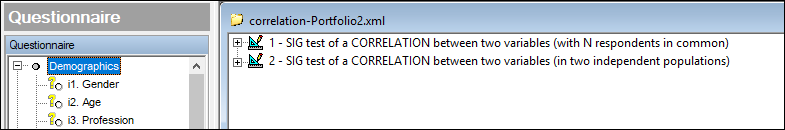Sig test of a correlation between two variables (with N respondents in common)

The formula is: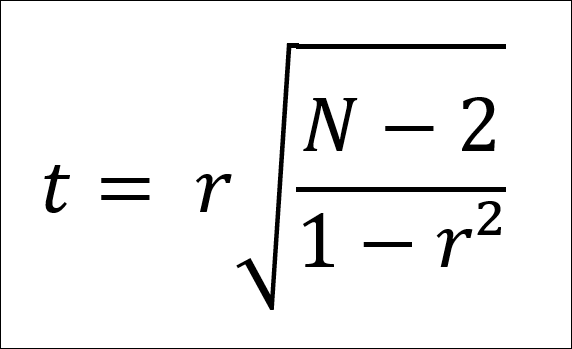To test whether a correlation r between 2 variables with N respondents in common is significantly different from zero.

t is the sigma value

• If t > 1.645, significance at 90%
• If t > 1.960, significance at 95%
• If t > 2.576, significance at 99%

The test follows a reduced normal centred law on which a Student's test can be applied.

An example correlation table definition is shown below: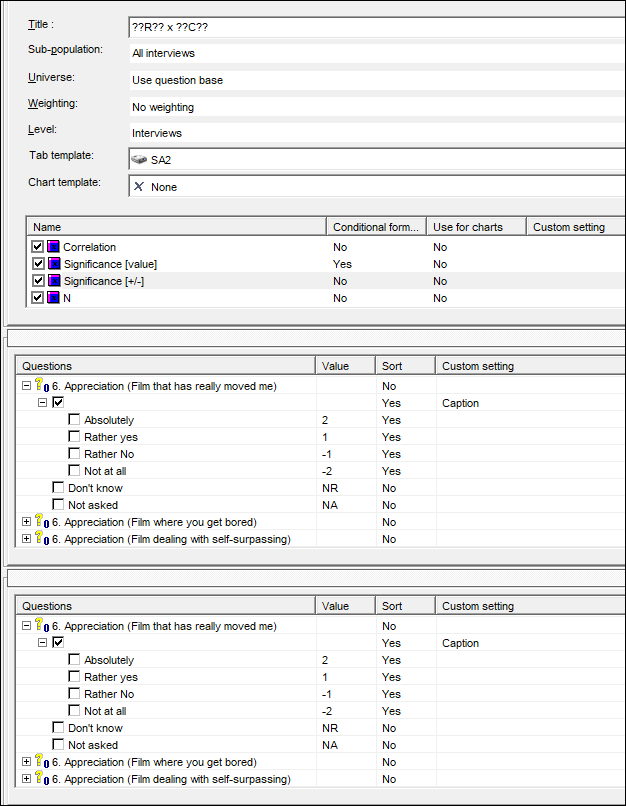The result: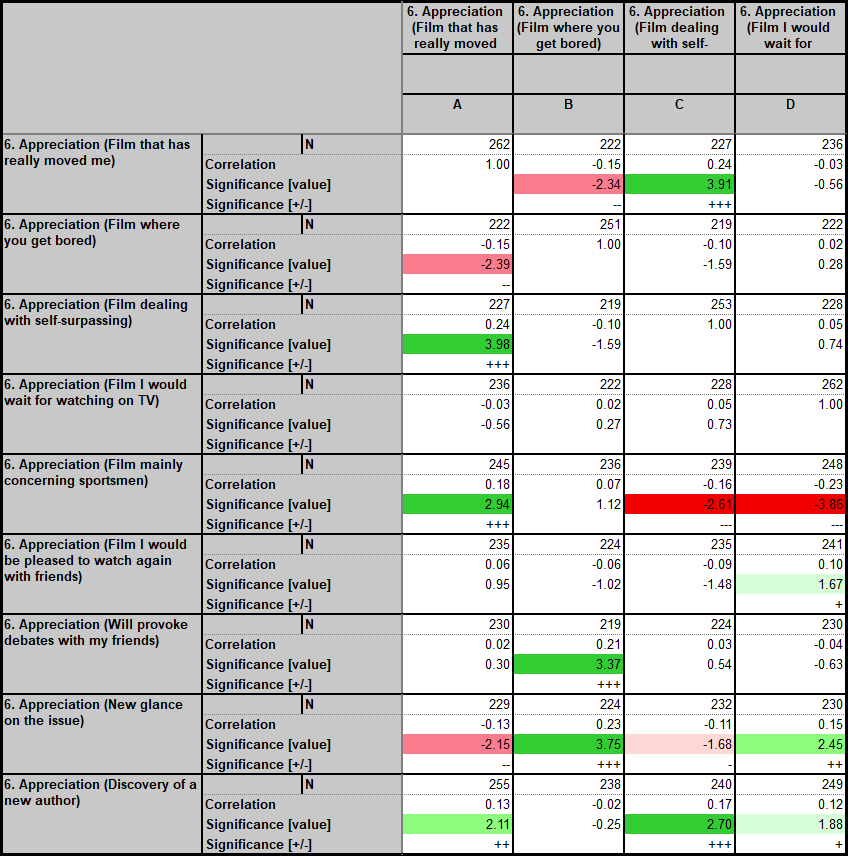The Significance [value] calculation is done using calculation arithmetic:

```Dim r1 = CurrentTable.GetCell(CurrentCell.X,CurrentCell.Y-1).Value
Dim n1 = CurrentCell.Row.Question.Data.FilterbyXY().Size()

Dim Denom1 = 1 - Pow(r1,2)
Dim tValue = r1 * (Pow( ( (n1-2) / Denom1 ) , 0.5))

If r1 <> 1 Then
Return tValue
Else
Return nr
Endif
```

The conditional formatting is set on this calculation as follows: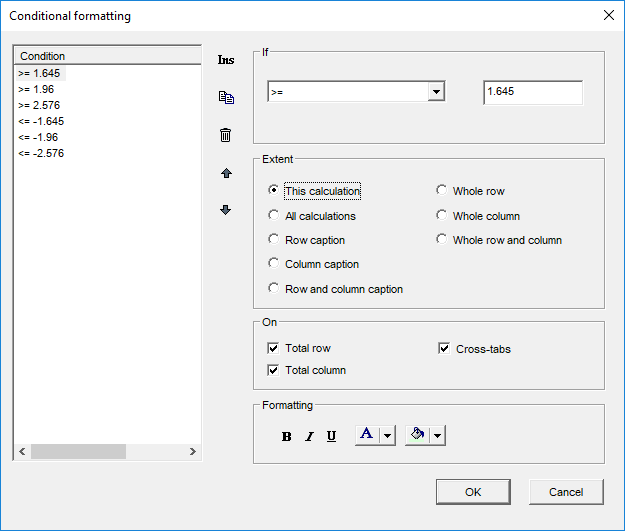The Significance [+/-] calculation can then be done using calculation arithmetic:

```Dim r1 = CurrentTable.GetCell(CurrentCell.X,CurrentCell.Y-2).Value
Dim s1 = CurrentTable.GetCell(CurrentCell.X,CurrentCell.Y-1).Value
'+++
If ((s1 >= 1.645 and s1 < 1.96)) and r1<>1 Then
Return "+"
Endif
If ((s1 >= 1.96 and s1 < 2.576)) and r1<>1 Then
Return "++"
Endif
If (s1 >= 2.576) and r1<>1 Then
Return "+++"
Endif
'---
If ((s1 <= -1.645 and s1 > -1.96)) and r1<>1 Then
Return "-"
Endif
If ((s1 <= -1.96 and s1 > -2.576)) and r1<>1 Then
Return "--"
Endif
If (s1 <= -2.576) and r1<>1 Then
Return "---"
Endif```

If you just want the colouring to show the significance you can hide both these calculations and set the conditional formatting to take effect on 'All calculations'.

Sig test of a correlation between two variables (in two independent populations)

The formula is: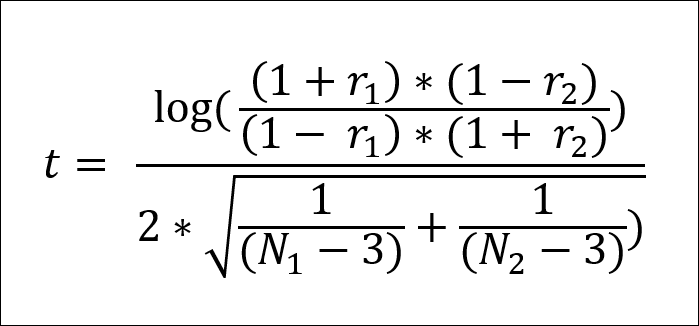Where r1 is the correlation between 2 variables on a sample size of N1 and r2 is the correlation between the same 2 variables on a second independent sample size of N2

again t is the sigma value

• If t > 1.645, significance at 90%
• If t > 1.960, significance at 95%
• If t > 2.576, significance at 99%

The test follows a reduced normal centred law on which a Student's test can be applied.

The table set-up is similar with the inbuilt Correlation and N (count) calculations used. However, in order to provide the independent sample columns we put the variable, i2. Age, in the edges and select the option break option shown below: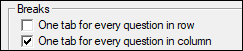The result: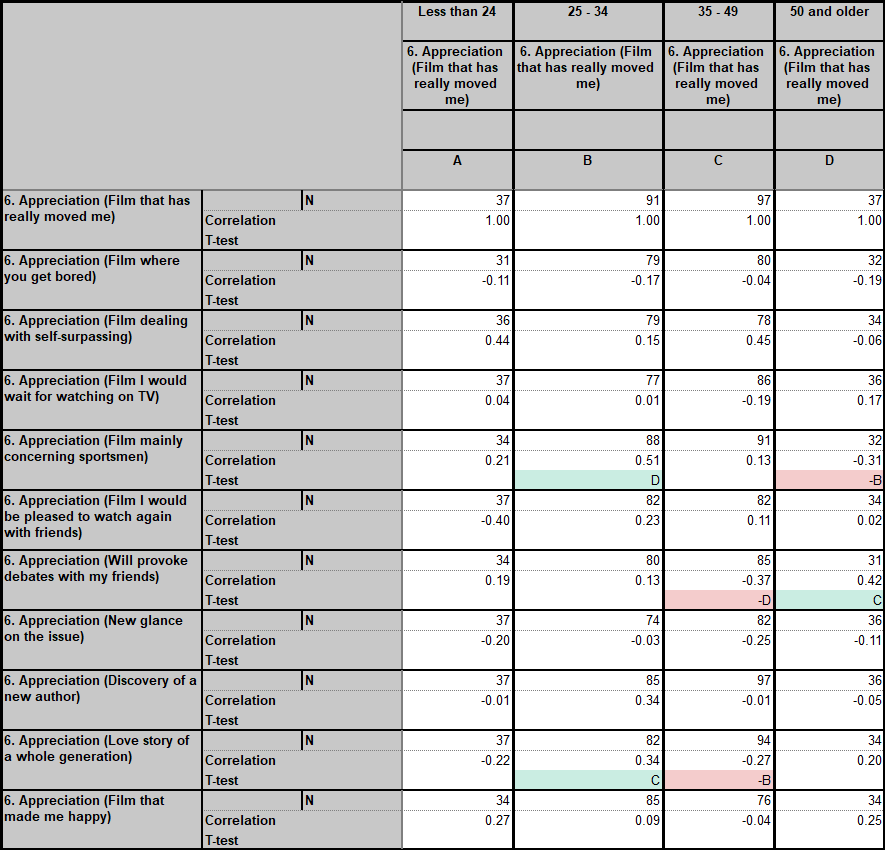The calculation which returns the col sig letters is T-Test.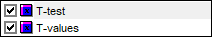The other calculation, T-values, returns the sigma values of the current column against all the others in an array. This can be useful for checking e.g.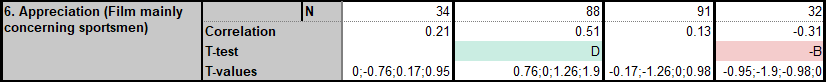The calculation arithmetic script for T-Test is:

```Dim N1 = CurrentCell.Row.Question.Data.FilterByXY().Size()
Dim R1 = CurrentTable.GetCell(CurrentCell.X,CurrentCell.Y-1).Value

Dim strColsig = ""
Dim i = 0

For i = currentTable.StartX to currentTable.MaxX

Dim N2 = CurrentCell.Row.Question.Data.FilterByXY(i).Size()
Dim R2 = CurrentTable.GetCell(i,CurrentCell.Y-1).Value

Dim dDenomP = ( ( (1+R1) * (1-R2) ) / ( (1-R1) * (1+R2) ) )
Dim dNumeratorP = ( ( 1 / (N1-3) ) + ( 1 / (N2-3) ) )
Dim dSigma = dDenomP.Log10() / ( 2 * ( dNumeratorP.Pow(0.5) ) )' The test is done at 90% and the letter is shown with a minus sign if significantly lower
If dSigma.Abs() > 1.65 and R1 > R2 Then
strColsig = strColsig + CurrentTable.GetColSigLetter(i,2)
ElseIf dSigma.Abs() > 1.65 and R2 > R1 Then
strColsig = strColsig + CurrentTable.GetColSigLetter(i,-2)
Endif

Next i
Return strColsig```

The conditional formatting this time is taken care of by a cleaning script (General tab > Settings > Sorting).

```' Conditional formatting
Dim i = 0
Dim j = 0
Dim k = 0
Dim h = 0

Dim arrColSigLetters = {""}
For i = currentTable.StartX to currentTable.MaxX
arrColSigLetters = arrColSigLetters + CurrentTable.GetColSigLetter(i)
Next i

arrColSigLetters = arrColSigLetters.RemoveAt(1)

For k = currentTable.StartX to currentTable.MaxX
For j = currentTable.StartY to currentTable.MaxY
For h = 1 to arrColSigLetters.Count
If Instr((arrColSigLetters[h]).ToString(), CurrentTable.GetCell(k,j).Text) and Instr("-", CurrentTable.GetCell(k,j).Text) Then
CurrentTable.GetCell(k,j).SetBackColor(244,204,204)
ElseIf Instr((arrColSigLetters[h]).ToString(), CurrentTable.GetCell(k,j).Text) Then
CurrentTable.GetCell(k,j).SetBackColor(202,237,226)
EndIf
Next h
Next j
Next k
```